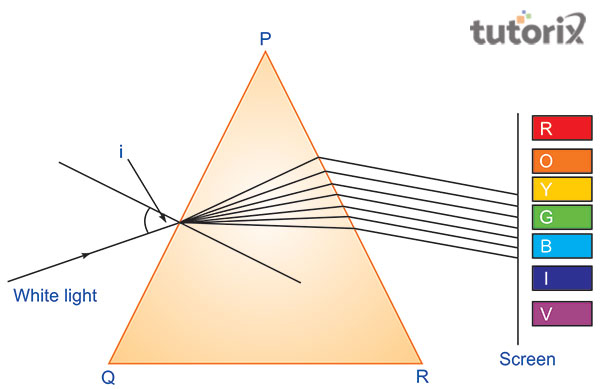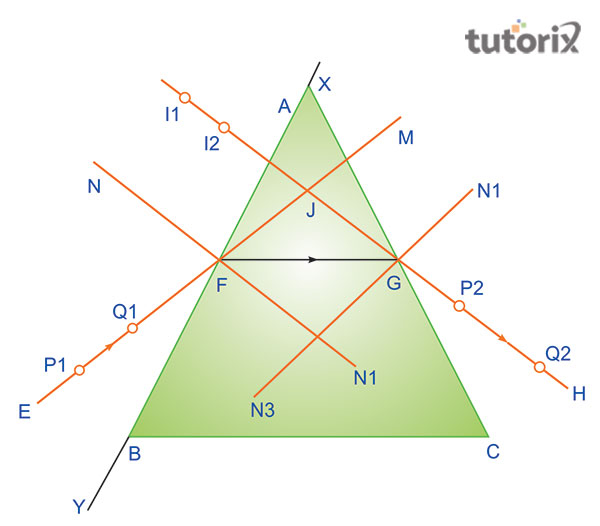# Tracing the path of the rays of light through a glass Prism

## Introduction

Identifying the path of the white light through a triangular prism is an important scientific experiment. It is used to exhibit the spectrum of visible light. Different types of light hold different colors when they pass through a triangular prism they are divided into multiple spectra and thus by this experiment is found that a mere white light possesses the different colors in it. This experiment was first done by Isaac Newton in 1665.

## Some significant facts of Triangular Prism

Triangular prism is generally a polyhedron that has two parallel bases. When a particular ray of light divided into two refractions while travelling through the prism, it separates in certain angle from the real path.

The angle of deviation relies on angle of prism, materialistic nature of the prism, and angle of incidence. Angle of deviation generally tends to decrease with the increase of the angle of increase. The minimum value of angle of deviation is named as angle of minimum deviation, for the prism. As opined by Nagare et al. (2019), reflected ray becomes parallel to the base of the prism under minimum deviation position.Figure 1: Prism and Light refraction

When the beam of light is reflected on one side of the surface of the prism it divides into seven colours. As opined by Hess et al. (2020), this particular phenomenon demonstrated by a prism is named dispersion of light. This collective band of the seven colours of the lights is known as spectrum.

Glass prism exhibits the various refractive indices which include the several color compounds of the white light. It happens because of the varying speed of each color. Thus, different colors come from the prism being different and thus becoming distinct (Smith & Karunadasa, 2018) White light spreads into VIBGYOR (Violet, Indigo, Blue, Green, Yellow, Orange, and Red) with Violet showing maximum deviation and red showing minimum deviation.

### Aim

The aim of the experiment is to identify path of rays of light by glass prism.

## Materials required

• Drawing pencil
• soft board
• a glass prism
• cello tape
• a few alpins
• 3 sheets of white paper
• Protractor
• Pencil, scale.

### ProcedureFigure 2: path of rays of light

The tracing of light through a triangular prism is an important scientific experiment and it also can be done by following the procedures mentioned below −

White paper is fixed on the drawing board with cello tape.

• Glass prism is placed in the middle of the paper. Boundary of the glass prism is marked as ABC and then the prism is removed.
• XY is then drawn on refracting surface (AB).
• Normal NFN1 is drawn perpendicular at F. A 30° angle is drawn by drawing line EF that acts as an incident ray to the normal.
• Glass prism is them positioned on ABC.
• Alpins Q1 and P1 are fixed on EF vertically by placing them at a distance of 6cm.
• Image of Q1 and P1 appears at I2 and I1 upon looking from refracting side AC.
• Alpins P2 and Q2 are then placed vertically. It is to be ensured that P2 and Q2 appear in straight line as I2 and I2.
• Both P2 and Q2 and P1 and Q1 move together if they are located on a straight line.
• Positions of P2, Q2, P1 and Q1 are marked after removing the prism.
• Point P2, Q2 is joined to produce P2Q2 ensuring that it meets on AC at G. thus HG represents emergent ray.
• F and G are joined together to denote path of refracted ray
• Join F and G. The line FG represents the path of the refracted ray.

## Observation

When the beam of light strikes the refracting surface, light ray bends towards normal after refraction (Ma et al. 2021). However, at the second refracting surface, the beam of light entering from air to glass bends away from the normal.

## Result

EFGH describes a certain path of the light through a prism and it is presented in the diagram.

## Precautions

The refracting face of glass prism needs to be smooth and transparent and should be free of broken age or are bubble. It should be triangular in shape. A sharp pencil is needed for drawing the boundary of prism as well as beams of lights. As opined by Zhdanov et al. (2018), the alpins should be shaped and needs to be fixed vertical to the plan of paper. 6m distance is needed between alpins. It gives the perfect accuracy to find the direction of the refracted ray and incident ray. The feet of alpins should be adjusted always and not the head. Pins must be circled and dotted. Angle of incidence must be from 30° to 60° (Taravati & Eleftheriades, 2021). The appropriated arrows essentially need to be drawn to indicate the direction of incident ray, emergent ray and refracted ray.

## Conclusion

Tracing of the light through a particular prism is very important as it helps to understand the different compounds of light that are invisible to the open eyes. This particular experiment shows that the mere white light possesses the different seven colors in it. These seven colors of the light are collectively known as spectrum.

## FAQs

Q1. What is a prism?

Prism refers to an optical object which has a transparent, polished and flat surface which refers the lights. A prism is general used to experiment the refection of light.

Q2. Which unit is used for refractive index?

Refractive unit doesn't nit possess any unit. This is because of the fact that it is the ratio between speed of light in particular medium and in vacuum.

Q3. What is meant by dispersion of light?

It refers to the dispersion of white light splitting into seven different colors. This phenomenon happens when the light travels through a lucid medium.

Q4. What occurs when the incident ray enters into a prism?

An incident ray generally bends to normal while passing through the prism. It happens because the ray changes its original path and becomes deviated.